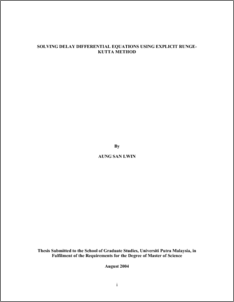# Solving Delay Differential Equations Using Explicit Runge-Kutta Method

## Citation

Aung, San Lwin (2004) Solving Delay Differential Equations Using Explicit Runge-Kutta Method. Masters thesis, Universiti Putra Malaysia.

## Abstract

Introduction to delay differential equations (DDEs) and their examples are presented. The General formulation of Explicit Runge-Kutta method when adapted to delay differential equations is described. Delay Differential Equations are solved by embedded Explicit Runge-Kutta method, which is more attractive from the practical point of view. Embedding technique is used to solve DDEs not just with single delay, but with multiple delays. The technique is also used to get the local truncation error which provides a basis for choosing the next stepsize for the integration. The delay terms are approximated using three techniques of interpolation, which are the divided difference interpolation, Hermite interpolation, and continuous extensions formula of the Runge-Kutta method itself. Numerical results of tested problems based on these interpolations are presented and compared. Finally, the stability properties of Explicit Runge-Kutta method when applied to DDEs using Lagrange interpolation, Hermite interpolation and continuous extensions Runge-Kutta formula are investigated and their stability regions are illustratedPreview
PDF
549697_FSAS_2004_37.pdfView Item